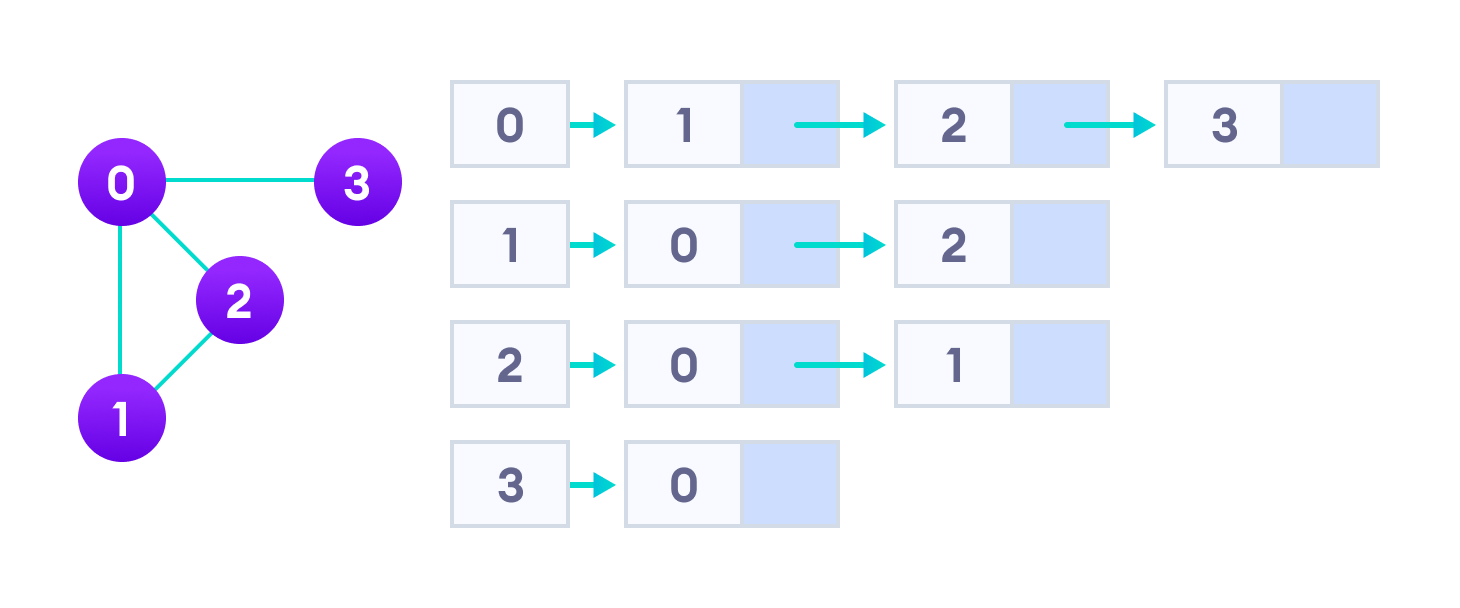Hits: 0

# Adjacency List

#### In this tutorial, you will learn what an adjacency list is. Also, you will find working examples of adjacency list in Python.

An adjacency list represents a graph as an array of linked lists.

The index of the array represents a vertex and each element in its linked list represents the other vertices that form an edge with the vertex.

## Adjacency List representation

A graph and its equivalent adjacency list representation are shown below.Adjacency List representation

An adjacency list is efficient in terms of storage because we only need to store the values for the edges. For a sparse graph with millions of vertices and edges, this can mean a lot of saved space.

## Adjacency List Structure

The simplest adjacency list needs a node data structure to store a vertex and a graph data structure to organize the nodes.

We stay close to the basic definition of a graph – a collection of vertices and edges `{V, E}`. For simplicity, we use an unlabeled graph as opposed to a labeled one i.e. the vertices are identified by their indices 0,1,2,3.

Let’s dig into the data structures at play here.

``````struct node
{
int vertex;
struct node* next;
};

struct Graph
{
int numVertices;
struct node** adjLists;
};``````

Don’t let the `struct node** adjLists` overwhelm you.

All we are saying is we want to store a pointer to `struct node*`. This is because we don’t know how many vertices the graph will have and so we cannot create an array of Linked Lists at compile time.

## Adjacency List C++

It is the same structure but by using the in-built list STL data structures of C++, we make the structure a bit cleaner. We are also able to abstract the details of the implementation.

``````class Graph
{
int numVertices;
list<int> *adjLists;

public:
Graph(int V);
void addEdge(int src, int dest);
};``````

## Adjacency List Java

We use Java Collections to store the Array of Linked Lists.

``````class Graph
{
private int numVertices;
private LinkedList<integer> adjLists[];
}``````

The type of LinkedList is determined by what data you want to store in it. For a labeled graph, you could store a dictionary instead of an Integer

## Adjacency List Python

There is a reason Python gets so much love. A simple dictionary of vertices and its edges is a sufficient representation of a graph. You can make the vertex itself as complex as you want.

``````graph = {'A': set(['B', 'C']),
'B': set(['A', 'D', 'E']),
'C': set(['A', 'F']),
'D': set(['B']),
'E': set(['B', 'F']),
'F': set(['C', 'E'])}``````

## Python Examples

``````/* Adjascency List representation in Python */

class AdjNode:
def __init__(self, value):
self.vertex = value
self.next = None

class Graph:
def __init__(self, num):
self.V = num
self.graph = [None] * self.V

/* Add edges */
def add_edge(self, s, d):
node = AdjNode(d)
node.next = self.graph[s]
self.graph[s] = node

node = AdjNode(s)
node.next = self.graph[d]
self.graph[d] = node

/* Print the graph */
def print_agraph(self):
for i in range(self.V):
print("Vertex " + str(i) + ":", end="")
temp = self.graph[i]
while temp:
print(" -> {}".format(temp.vertex), end="")
temp = temp.next
print(" n")

if __name__ == "__main__":
V = 5

# Create graph and edges
graph = Graph(V)
graph.add_edge(0, 1)
graph.add_edge(0, 2)
graph.add_edge(0, 3)
graph.add_edge(1, 2)

graph.print_agraph()``````

# Special 95% discount

## 2000+ Applied Machine Learning & Data Science Recipes

### Portfolio Projects for Aspiring Data Scientists: Tabular Text & Image Data Analytics as well as Time Series Forecasting in Python & R## Two Machine Learning Fields

There are two sides to machine learning:

• Practical Machine Learning:This is about querying databases, cleaning data, writing scripts to transform data and gluing algorithm and libraries together and writing custom code to squeeze reliable answers from data to satisfy difficult and ill defined questions. It’s the mess of reality.
• Theoretical Machine Learning: This is about math and abstraction and idealized scenarios and limits and beauty and informing what is possible. It is a whole lot neater and cleaner and removed from the mess of reality.

Data Science Resources: Data Science Recipes and Applied Machine Learning Recipes

Introduction to Applied Machine Learning & Data Science for Beginners, Business Analysts, Students, Researchers and Freelancers with Python & R Codes @ Western Australian Center for Applied Machine Learning & Data Science (WACAMLDS) !!!

Latest end-to-end Learn by Coding Recipes in Project-Based Learning:

Applied Statistics with R for Beginners and Business Professionals

Data Science and Machine Learning Projects in Python: Tabular Data Analytics

Data Science and Machine Learning Projects in R: Tabular Data Analytics

Python Machine Learning & Data Science Recipes: Learn by Coding

R Machine Learning & Data Science Recipes: Learn by Coding

Comparing Different Machine Learning Algorithms in Python for Classification (FREE)

`Disclaimer: The information and code presented within this recipe/tutorial is only for educational and coaching purposes for beginners and developers. Anyone can practice and apply the recipe/tutorial presented here, but the reader is taking full responsibility for his/her actions. The author (content curator) of this recipe (code / program) has made every effort to ensure the accuracy of the information was correct at time of publication. The author (content curator) does not assume and hereby disclaims any liability to any party for any loss, damage, or disruption caused by errors or omissions, whether such errors or omissions result from accident, negligence, or any other cause. The information presented here could also be found in public knowledge domains.  `